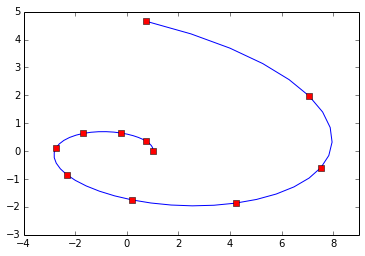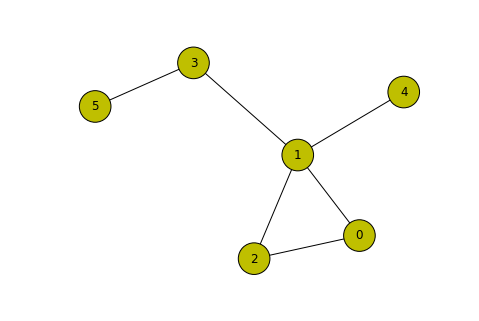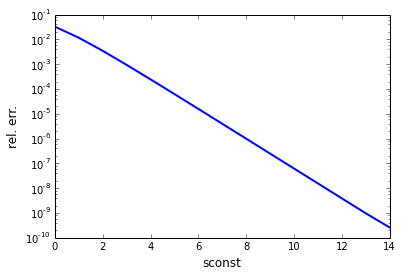# The Matrix Exponential¶

This notebook gives a brief introduction to the matrix exponential, an interesting function which can be found within many areas of applied mathematics. After defining the function we will introduce some applications and algorithms before giving links to currently available software and further information.

## Definition¶

In :
%matplotlib inline
import numpy as np
import scipy as sp
import scipy.linalg as la
import matplotlib.pyplot as plt


The matrix exponential is a direct generalisation of the corresponding scalar function. Remember that the Taylor series expansion for $\exp(x)$ is $$\exp(x) = \sum_{k = 0}^\infty \frac{x^k}{k!}.$$ We can apply this to square matrices using matrix multiplcation: $$\exp(A) = \sum_{k = 0}^\infty \frac{A^k}{k!}.$$

Two of the most useful properties of the matrix exponential (see  for more) are that $$\exp((a + b)A) = \exp(aA)\exp(bA),$$ and when two matrices $A$ and $B$ satisfy $AB = BA$, $$\exp(A + B) = \exp(A)\exp(B).$$

A thorough treatment of the theory behind the matrix exponential (and matrix functions in general) can be found in .

## Applications¶

The matrix exponential has a wide variety of applications in areas including cancer research  (which also uses the derivative of the exponential), nuclear burnup equations , and computer graphics . We'll cover two here: solving systems of linear differential equations and finding the important nodes in a complex network.

### Differential Equations¶

One major attraction of the matrix exponential is the fact that it gives the explicit solution to the differential equation $$y'(t) = A y(t),\qquad y(0) = y_0,$$ for $y, y_0 \in \mathbb{C}^n$ and $A \in \mathbb{C}^{n \times n}$. The solution is $$y(t) = \exp(At) y_0.$$

Knowing this we can compute the solution $y(t)$ at any time point directly without using a time-stepping scheme such as the Euler method, for example. Let's do a simple example showing this for the differential equation $$y'(t) = \begin{bmatrix}1 & -20\\3 & 4\end{bmatrix} y(t),\qquad y(0) = \begin{bmatrix}1\\0\end{bmatrix},\qquad t \in [0, 1].$$

In :
import scipy.integrate as sint

A = np.array([[1, -20],
[3, 4]], dtype=np.double)

def yprime(y, t):
return sp.dot(A, y)

t = np.linspace(0, 1, 51)
yzero = np.array([1, 0])

# Solve equation using odeint
y = sint.odeint(yprime, yzero, t) # Calls the Fortran library odeint
plt.plot(y[:, 0], y[:, 1]) # Blue line is y(t) for t in [0, 1]

plt.xlim([-4, 9])
plt.ylim([-3, 5])

# Solve for 10 t values using the exponential
tvals = np.linspace(0, 1, 11)

for tval in tvals:
yval = sp.dot(la.expm(A*tval), yzero)
plt.plot(yval, yval, 'rs') # Red squares are evaluated via the matrix exponentialFor our relatively simple ODE both methods perform well and, since the matrix exponential is more expensive to compute, we would probably opt to use scipy.integrate.odeint. For solving more complex differential equations the exponential forms the basis of a class of methods called exponential integrators . Some more detail on these methods can be found in , for example. Note that in practice we would calculate $\exp(A)b$ without first computing $\exp(A)$ as this is cheaper. We could compute $\exp(A)b$ directly by using scipy.sparse.linalg.expm_multiply, which implements the recent algorithm from .

### Important Nodes in a Network¶

Another recent application of the exponential is in finding the most influential nodes of a network. A network is a set of nodes connected by edges. We can represent a network by its adjacency matrix: we set $A_{ij} = 1$ if node $i$ is connected to node $j$ and $A_{ij} = 0$ otherwise. For example take the following network.

In :
import networkx as nx

Adj = np.array([[0, 1, 1, 0, 0, 0],
[1, 0, 1, 1, 1, 0],
[1, 1, 0, 0, 0, 0],
[0, 1, 0, 0, 0, 1],
[0, 1, 0, 0, 0, 0],
[0, 0, 0, 1, 0, 0]])

nx.draw(G, node_color='y', node_size=1000)One measure of the importance of each node is its centrality , , . It is well known that the number of walks of length $k$ from node $i$ to node $j$ is given by $A^k_{ij}$. We can add up all these contributions to obtain the centrality of node $i$ as $$c(i) = \alpha_1 A_{ii} + \alpha_2 A^2_{ii} + \alpha_3 A^3_{ii} + \cdots,$$ where the coefficients $\alpha_k$ remain to be chosen. Typically we assume that shorter walks are more important than longer ones so $\alpha_k \ge \alpha_{k+1}$. There are a number of proposed formulae for $\alpha_k$ but taking $\alpha_k = \frac{1}{k!}$ we obtain $c(i) = \exp(A)$.

Looking at our network above we might expect node 1 to be the most important: it has the highest degree and links nodes 3, 4, and 5 to nodes 0 and 2. Let's work out the centrality of each node and rank them in order of importance.

In :
centralities = np.diag(la.expm(np.array(Adj, dtype=np.double)))
nodeorder = np.argsort(centralities)[::-1]

print np.array([nodeorder, centralities[nodeorder]])

# Note: This is already built into networkx using the following command
# print nx.communicability_centrality_exp(G)

[[ 1.          0.          2.          3.          4.          5.        ]
[ 4.44723536  2.86427609  2.86427609  2.36018456  1.71615913  1.59432922]]


As we expected node 1 was the most important, followed by nodes 0 and 2 which have identical centralities since switching the two nodes wouldn't change the appearance of the graph.

## Computing the Exponential¶

In this section we will give a basic algorithm to compute the matrix exponential. We will use the Taylor series (from the first section), which converges for every $A$ but the convergence can be very slow when $\|A\|$ is large. To speed it up we can use the relationship $$\exp\left(\frac{A}{p}\right)^{p} = \exp(A)$$ repeatedly with $p = 2$ to make $\|A/2^s\|$ small before applying the Taylor series and squaring the result $s$ times. This is the basis of the scaling and squaring algorithm.

A number of potential methods for computing the exponential are described in detail within , .

In :
sconst = 1

taylordegree = 15 # Use order 15 Taylor approximation
s = np.ceil(sp.log2(la.norm(A))) + sconst # Find s such that norm(A/2^s) is small.
X = A/(2**s)
eX = np.eye(np.shape(A))
for k in range(taylordegree): # Compute the Taylor series
eX = eX + X/sp.misc.factorial(k+1)
X = sp.dot(X, X)

for k in range(np.int64(s)):
eX = sp.dot(eX, eX) # Do the squaring phase of the algorithm

return eX

#Let's test it against la.expm
A = np.random.randn(4, 4)
Eexact = la.expm(A)
print la.norm(E1 - Eexact)/la.norm(Eexact) # Relative error

0.0116677518269


Our algorithm worked but the relative error compared to scipy.linalg.expm is quite poor. If we increase sconst then $\|X\|$ will be much smaller and the Taylor series will be more accurate.

In :
relerrs = np.zeros(15)
for sconst in range(15):
relerrs[sconst] = la.norm(E - Eexact)/la.norm(Eexact)

plt.plot(relerrs, color='blue', lw=2)
plt.yscale('log')
plt.xlabel('sconst', fontsize=12)
plt.ylabel('rel. err.', fontsize=12)

Out:
<matplotlib.text.Text at 0x4441fd0>This helps a lot but we are still not getting the same accuracy as scipy.linalg.expm.

The extra accuracy of scipy.linalg.expm is because it implements the algorithm from  which:

• analyses the backward error of the function.
• uses cost analysis to perform the least possible number of matrix multiplications.

## Available Software¶

There is a lot of software available for computing the matrix exponential.

In Python:

In MATLAB:

• expm - built-in function evaluating $\exp(A)$ (currently using an older algorithm than SciPy).
• expm_new - implements algorithms from .
• expmv - implements algorithms from .
• expm_frechet_pade - computes the derivative of $\exp(A)$ as explained in .
• expm_frechet_quad - computes the derivative of $\exp(A)$ as explained in .
• expm_cond - computes the condition number of $\exp(A)$ in the Frobenius norm as explained in .
• expokit - computes $\exp(A)$ for small dense $A$ or $\exp(A)B$ for sparse $A$.

NAG Library (Fortran but callable in MATLAB, Python etc.):

• F01(E/F)CF - Real / Complex matrix exponential using .
• F01(E/F)DF - computes the matrix exponential of a real symmetric / complex Hermitian matrix.
• F01(G/H)AF - Real / Complex versions of $\exp(A)B$ using .
• F01(J/K)AF - estimates the condition number of the matrix exponential for real / complex input using .

## Further Information¶

Some further information not directly referenced here can be found at:

• Cleve's Corner - discusses the accuracy of computing $\exp(A)$.
• Wikipedia - a more detailed introduction to the matrix exponential.
• Software Catalogue - A catalogue of available matrix function software (currently version 1.0)
• Nick Higham's Blog - An short course on matrix functions (video lectures and written notes)

 - A. H. Al-Mohy and N. J. Higham, Computing the Frèchet Derivative of the Matrix Exponential, with an Application to Condition Number Estimation, SIAM J. Matrix Anal. Appl., 30:1639-1657, 2009.

 - A. H. Al-Mohy and N. J. Higham, A New Scaling and Squaring Algorithm for the Matrix Exponential, SIAM J. Matrix Anal. Appl., 31(3):970-989, 2009.

 - A. H. Al-Mohy and N. J. Higham, Computing the Action of the Matrix Exponential, with an Application to Exponential Integrators, SIAM J. Sci. Comput., 32:488-511, 2011.

 - Ernesto Estrada and N. Hatano, Communicability in Complex Networks, Phys. Rev. E., 77(3):036111, 2008.

 - Ernesto Estrada and D. J. Higham, Network Properties Revealed Through Matrix Functions, SIAM Rev. 52:696-714, 2010.

 - Ernesto Estrada, D. J. Higham, and N. Hatano, Communicability Betweeness in Complex Networks, Physica A: Statistical Mechanics and its Applications, 388:764-774, 2009.

 - Marlis Hochbruch and Alexander Osterman, Exponential Integrators, Acta Numerica, x:209-286, 2009.

 - C. B. Moler and C. F. Van-Loan, Nineteen Dubious Ways to Compute the Exponential of a Matrix, </i>SIAM Rev.</i>, 20:801-836, 1978.

 - C. B. Moler and C. F. Van-Loan, Nineteen Dubious Ways to Compute the Exponential of a Matrix, Twenty-Five Years Later, SIAM Rev., 45:3-49, 2003.

 - B. Garcìa-Mora, C. Santamarìa, G. Rubio, and J. L. Pontones, Computing Survival Functions of the Sum of Two Independent Markov Processes. An Application to Bladder Carcinoma Treatment, Int. Journal of Computer Mathematics, 91(2):209-220, 2014.

 - N. J. Higham - Functions of Matrices: Theory and Computation, Society for Industrial and Applied Mathematics, Philadelphia, PA, USA, 2008, ISBN: 978-0-898716-46-7, xx+425 pp.

 - Maria Pusa and Jaakko Leppǎnen Computing the Matrix Exponential in Burnup Calculations, Nuclear Science and Engineering, 164(2):140-150, 2010.

 - Jarek Rossignac and Àlvar Vinucua, Steady Affine Motions and Morphs, ACM Trans. Graph., 30(5):Article 116, 16 pages, 2011.